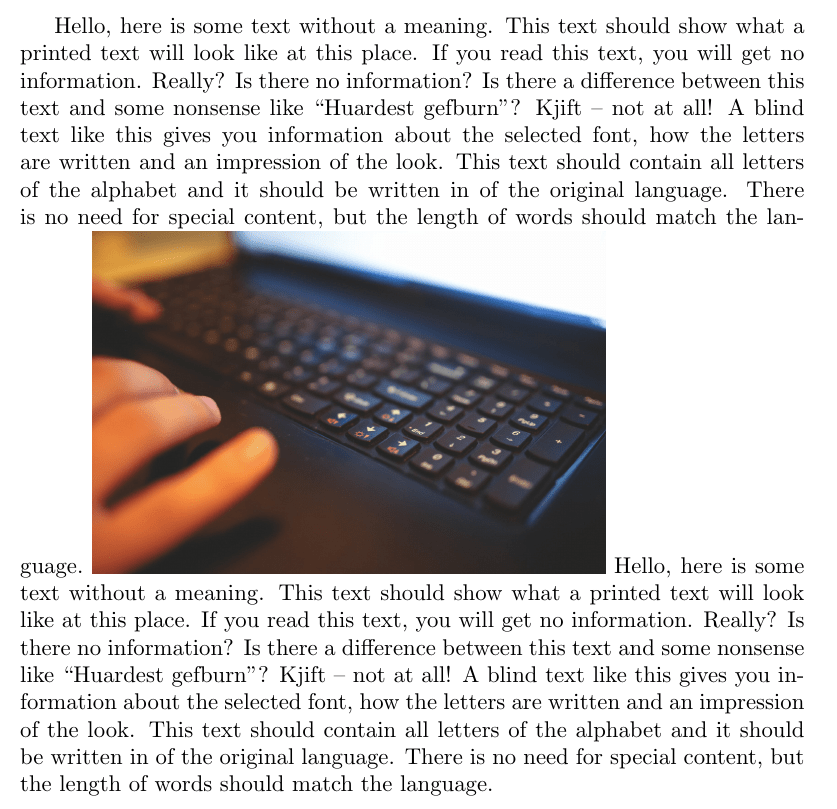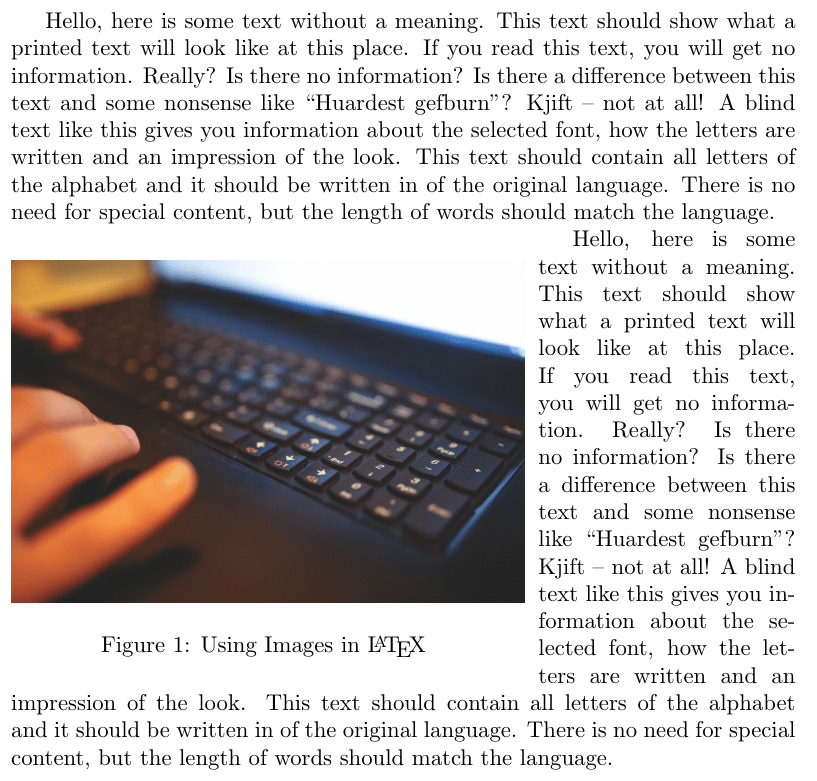# Insert Images into a LaTeX Document

We have all heard that a picture is worth a thousand words. Images help people to understand concretely what a writer is trying to communicate with text. In this post, we will look at how to include images inside documents prepared with LaTeX.Basic Example

One way to include an image is to use the “graphicx” package and to set the path for where the image is using the “\garphicspath” declaration in the preamble of a LaTeX document. Below is an example. Included in the example is the “babel” and “blindtext” packages to create some filler text.

\documentclass{article}
\usepackage[english]{babel}
\usepackage{blindtext}
\usepackage{graphicx}
\graphicspath{ {PUT THE PATH HERE} }
\begin{document}
\blindtext

\includegraphics[scale=.1]{1.jpg}

\blindtext
\end{document}Inside the actual document we use the following declaration

\includegraphics[scale=.1]{1.jpg}

“\includegraphics” is the declaration. The “scale” argument reduces the size of the image. The information in the curly braces is the name of the actual file. You can see that our print out is rather ugly and needs refinement.

One thing our picture needs is a caption that describes what it is. This can be done by first creating a figure environment, placing the “\includegraphics” declaration inside it, and using the “\caption” declration. Below is an example. We will also center the image for aesthetic reasons as well.

\documentclass{article}
\usepackage[english]{babel}
\usepackage{blindtext}
\usepackage{graphicx}
\graphicspath{ {PUT THE PATH HERE} }
\begin{document}
\blindtext

\begin{figure}
\centering
\includegraphics[scale=.1]{1.jpg}
\caption{Using Images in \LaTeX}
\end{figure}

\blindtext
\end{document}We created a figure environment added our image and type a caption. LaTeX automatically added “Figure 1” to the image. In addition,  you can see that the picture moved to the top of the page. This is because environments are able to float to the best position on a page as determined by calculations made by LaTeX.

If you want the image to appear in a particular place you can add the optional arguments h,t,b,p next to the “\begin{figure}” declaration. h =  here, t = top, b = bottom, and p = separate page.

To get rid of floating use the package called “capt-of”  and the declaration “\captionof{figure or table}{name here}}”. This will freeze the image in place so that it does not move all over the place as you add content to your document. Below is the same example but using the “capt-of” package.

\documentclass{article}
\usepackage[english]{babel}
\usepackage{blindtext}
\usepackage{graphicx}
\graphicspath{ {PUT PATH HERE} }
\usepackage{capt-of}
\begin{document}
\blindtext

\begin{center}
\includegraphics[scale=.1]{1.jpg}
\captionof{figure}{Using Images in \LaTeX}
\end{center}

\blindtext
\end{document}This is almost like our first example except now we have a caption. We did have to create a center environment but this type of environment does not float.

Wrapping Figures

The last example is wrapping text around a figure. For this, you need the “wrapfig” package and you need to create an environment with the “Wrapfigure” command. You also must indicate where the figure should be to the left (l),  center (c), or to the right (r). Lastly, you need to indicate the width of the figure. Below is the code followed by the results.

\documentclass{article}
\usepackage[english]{babel}
\usepackage{blindtext}
\usepackage{graphicx}
\usepackage{wrapfig}
\begin{document}
\blindtext

\begin{wrapfigure}{r}{7.8cm}
\includegraphics[scale=.1]{1.jpg}
\caption{Using Images in \LaTeX}
\end{wrapfigure}

\blindtext
\end{document}In the example above, we moved the image to the left. For the width, you have to guess several times so that all of the text appears next to the figure rather than behind it.

Conclusion

This post provided several practical ways to include images in a LaTeX document. With this amount of control, you are able to make sophisticated documents that are consistently reproduced.